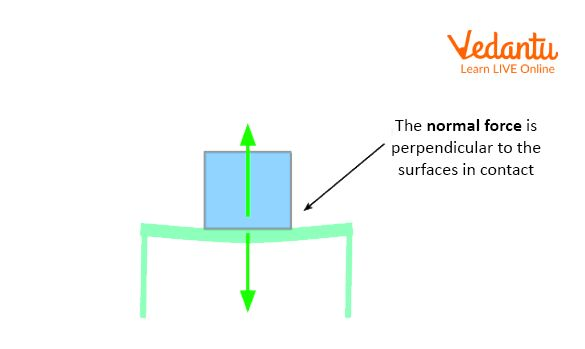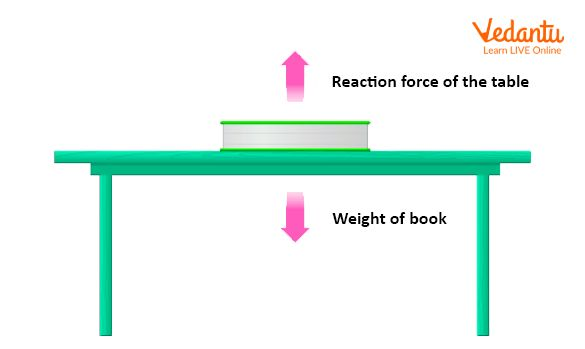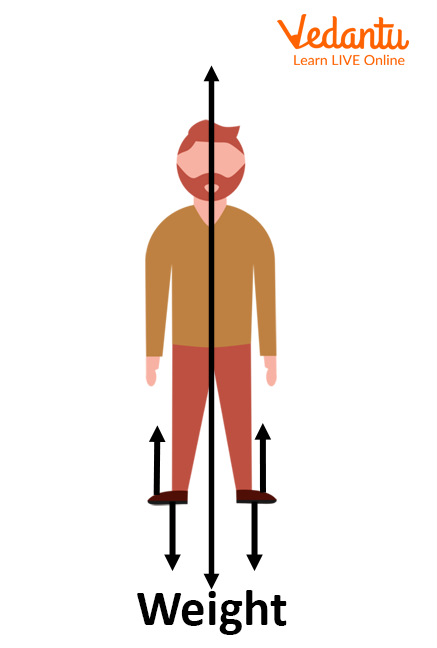Courses
Courses for Kids
Free study material
Offline Centres
More

# Tension, Normal And Spring Forces - JEE Important TopicLast updated date: 27th Nov 2023
Total views: 21k
Views today: 1.21k## What is Force?

In Physics, a force is any interaction that, when unopposed, will change the motion of an object. A force can cause an object with mass to change its velocity (which includes beginning moving from a state of rest), i.e., to accelerate.

Force can also be described by intuitive concepts such as a push or pull. A force has both magnitude and direction, making it a vector quantity. It is measured in the SI unit of newtons and represented by the symbol F.

The original form of Newton's second law states that the net force acting upon an object is equal to the rate of change of its momentum with time. If the mass of the object is constant, this law implies that the acceleration of an object is directly proportional to the net force acting on it and inversely proportional to its mass. The relationship between acceleration and the applied force is often expressed using Newton's third law which states that for every action, there is always an equal but opposite reaction.

## What is Normal Force?

In Physics, the normal force is the force that a surface exerts on an object that is in contact with it. The normal force is perpendicular to the surface and prevents the object from penetrating the surface. It is also sometimes called the "normal reaction" or "reaction force".Normal Force

The normal force arises from electrostatic interactions between atoms in the object and atoms on the surface. When two objects are brought into contact, their surfaces push against each other, preventing them from penetrating each other. The normal force is what keeps your hand from going through a table when you rest it on the table's surface.

The normal force can also be thought of as the force that a spring exerts on an object when the spring is compressed or stretched. For example, when you sit on a chair, your weight stretches out the springs in the chair, and they push back against you with a restoring force. This restoring force is due to the elasticity of the springs and it opposes your weight, keeping you from falling through the chair.

The magnitude of the normal force depends on how much pressure there is between the two surfaces in contact. If there is more pressure, then there will be a larger normal force. For example, if you lean against a wall, there will be more pressure between your body and the wall than if you were just resting your hand on a tabletop. This increased pressure results in a larger than normal force exerted by the wall on your body.

The normal force is the force that a surface exerts on an object that is in contact with it. The normal force can be calculated using the following formula:

Normal Force = Mass x Gravity

where

Mass is the mass of the object.

Gravity is the acceleration due to gravity 9.8 m/s2

Examples

• A book resting on a table. The weight of the book is pushing down on the table, and the table is pushing back up on the book with an equal force. This is an example of the normal force.Table Resting on Table

• A person standing on the ground. The person's weight is pushing down on the ground, and the ground is pushing back up with an equal force. This is an example of the normal force.Person Standing on Gound

## What is the Tension Force?

A tension force is a force that pulls on an object from two opposite directions. It is also known as a "tension" or "tensile" force. The magnitude of the tension force is equal to the sum of the forces pulling on the object from each direction.

The tension force formula is used to calculate the force exerted by a string or rope when it is pulled tight. This force is also known as the pulling force. The formula for tension force is:

Tension Force (N) = $Mass(kg)\times Acceleration(m/{{s}^{2}})$

where

Mass is the mass of the object being accelerated.

Acceleration is the acceleration of the object due to the applied force.

Examples

• The tension force is the force that pulls two objects together. It is the force that keeps your shoes tied to your feet and prevents them from falling off.

• The tension force also keeps the clothes on your body from falling off. It is the force that makes it possible for you to walk without your pants falling down.

• The tension force is also responsible for keeping the paper in a stack together. If there was no tension force, each sheet of paper would just fall on top of the other and create a big mess.

• Tension forces are also at work whenever you stretch a rubber band or string. The more you stretch it, the greater the tension force becomes.

## Spring Force

Spring forces are those that act on an object when it is compressed or stretched. The force exerted by a spring is proportional to the amount of compression or stretching. In other words, the more you compress or stretch a spring, the greater the force it exerts.

When an object is at rest on a surface, the force of gravity acting on the object is counteracted by the normal force exerted by the surface. However, when an object is compressed or stretched, it experiences a force that opposes its motion. This force is known as a spring force.

The formula for calculating the spring force is $F=-kx$

where F is the spring force (in Newtons), k is the spring constant (in Newtons per metre), and x is the displacement of the object from its equilibrium position (in metres).

• A person jumping on a trampoline.

• A coiled-up spring is being released.

• A cat stretches its back.

• A person bouncing on a pogo stick.

## Summary

In the article, we discussed the force concepts. Let’s summarise the article with the key points: A force is an external agent capable of changing the state of rest or motion of a particular body. It has a magnitude and a direction. The normal force is the force that a surface exerts on an object that is in contact with it. A tension force is a force that pulls on an object from two opposite directions. It is also known as a "tension" or "tensile" force. Spring forces are those that act on an object when it is compressed or stretched. The force exerted by a spring is proportional to the amount of compression or stretching. In other words, the more you compress or stretch a spring, the greater the force it exerts.

## FAQs on Tension, Normal And Spring Forces - JEE Important Topic

1. Mention a few effects of force.

The Force has different effects, and here are some of them.

• Force can make a body that is at rest move.

• It can stop a moving body or slow it down.

• It can increase the speed of a moving body.

• It can also change the direction of a moving body along with its shape and size.

2. Is tension force the same as spring force?

The tension force is directed along the length of the wire and pulls equally on the objects on the opposite ends of the wire. The spring force is the force exerted by a compressed or stretched spring upon any object that is attached to it.